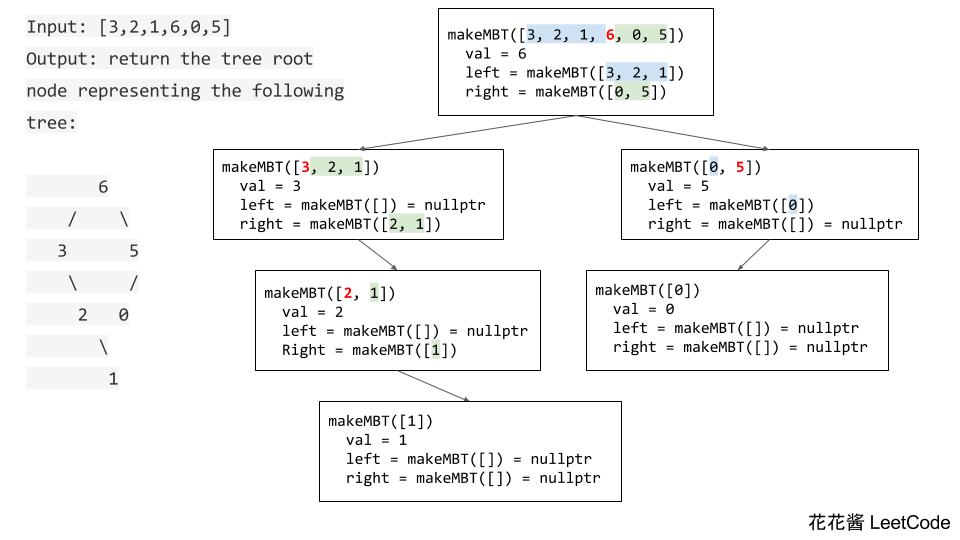# Problem

https://leetcode.com/problems/arithmetic-slices/description/

A sequence of number is called arithmetic if it consists of at least three elements and if the difference between any two consecutive elements is the same.

For example, these are arithmetic sequence:

1, 3, 5, 7, 9
7, 7, 7, 7
3, -1, -5, -9

The following sequence is not arithmetic.

1, 1, 2, 5, 7

A zero-indexed array A consisting of N numbers is given. A slice of that array is any pair of integers (P, Q) such that 0 <= P < Q < N.

A slice (P, Q) of array A is called arithmetic if the sequence:
A[P], A[p + 1], …, A[Q – 1], A[Q] is arithmetic. In particular, this means that P + 1 < Q.

The function should return the number of arithmetic slices in the array A.

## Example:

A = [1, 2, 3, 4]

return: 3, for 3 arithmetic slices in A: [1, 2, 3], [2, 3, 4] and [1, 2, 3, 4] itself.

# Solution 0: Reduction

Reduce the problem to # of all 1 sub arrays.

B[i – 2] = is_slice(A[i], A[i+1], A[i+2])

Time Complexity: O(n)

Space Complexity: O(n)

# Solution 1: Combined

C++

Time complexity: O(n)

Space complexity: O(1)

# Related Problems:

We are given an array A of positive integers, and two positive integers L and R (L <= R).

Return the number of (contiguous, non-empty) subarrays such that the value of the maximum array element in that subarray is at least L and at most R.

Solution 1:

C++

Solution 2: One pass

C++

Given an integer array with no duplicates. A maximum tree building on this array is defined as follow:

1. The root is the maximum number in the array.
2. The left subtree is the maximum tree constructed from left part subarray divided by the maximum number.
3. The right subtree is the maximum tree constructed from right part subarray divided by the maximum number.

Construct the maximum tree by the given array and output the root node of this tree.

Example 1:

Idea:

RecursionSolution:

With copy

Time complexity: O(nlogn) ~ O(n^2)

Space complexity: O(nlogn) ~ O(n^2)

running time 79ms

Without copy

Time complexity: O(nlogn) ~ O(n^2)

Space complexity: O(logn) ~ O(n)

running time 66ms

Mission News Theme by Compete Themes.